Search

Matrix Equality (page 3 of 3)

Sections: Augmented & coefficient matrices / Matrix size, Matrix notation & types, Matrix equalityFor two matrices to be equal, they must be of the same size and have all the same entries in the same places. For instance, suppose you have the following two matrices: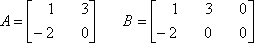These matrices cannot be the same, since they are not the same size. Even if A and B are the following two matrices: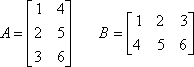...they are still not the same. Yes, A and B each have six entries, and the entries are even the same numbers, but that is not enough for matrices. A is a 3 × 2 matrix and B is a 2 × 3 matrix, and, for matrices, 3 × 2 does not equal 2 × 3! It doesn't matter if A and B have the same number of entries or even the same numbers as entries. Unless A and B are the same size and the same shape and have the same values in exactly the same places, they are not equal.

This property of matrix equality can be turned into homework questions. You will be given two matrices, and you will be told that they are equal. You will need to use this equality to solve for the values of variables.

• Given that the following matrices are equal, find the values of x and y.
•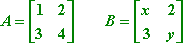For A and B to be equal, they must have the same size and shape (which they do; they're each 2 × 2 matrices) and they must have the same values in the same spots. Then a1,1 must equal b1,1, a1,2 must equal b1,2, and so forth. The entries a1,2 and a2,1 are clearly equal, respectively, to entries b1,2 and b2,1 "by inspection" (that is, "just by looking at them"). But a1,1 = 1 is not obviously equal to b1,1 = x. For A to equal B, I must have a1,1 = b1,1, so it must be that 1 = x. Similarly, I must have a2,2 = b2,2, so then 4 must equal y. Then the solution is:

• Given that the following matrices are equal, find the values of x, y, and z.
•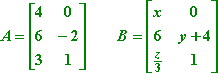To have A = B, I must have all entries equal. That is I must have a1,1 = b1,1, a1,2 = b1,2, a2,1 = b2,1, and so forth. In particular, I must have:

4 = x
2 = y + 4

3 = z/3

...as you can see from the highlighted matrices: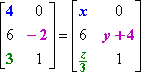Solving these three equations, I get:

x = 4, y = 6, and z = 9.

Don't let matrices scare you. Yes, they're different from what you're used to, but they're not so bad (at least not until you try to multiply them, but that's another lesson for another time).

<< Previous  Top  |  1 | 2 | 3  |  Return to Index

 Cite this article as: Stapel, Elizabeth. "Matrix Equality." Purplemath. Available from     https://www.purplemath.com/modules/matrices3.htm. Accessed [Date] [Month] 2016

Study Skills Survey

Tutoring from Purplemath
Find a local math tutor